The VARMAX Procedure

OUT= Data Set

The OUT= data set contains the forecast values produced by the OUTPUT statement. The following output variables can be created:

• the BY variables

• the ID variable

• the MODEL statement dependent (endogenous) variables. These variables contain the actual values from the input data set.

• FORi, numeric variables that contain the forecasts. The FORi variables contain the forecasts for the ith endogenous variable in the MODEL statement list. Forecasts are one-step-ahead predictions until the end of the data or until the observation specified by the BACK= option. Multistep forecasts can be computed after that point based on the LEAD= option.

• RESi, numeric variables that contain the residual for the forecast of the ith endogenous variable in the MODEL statement list. For multistep forecast observations, the actual values are missing and the RESi variables contain missing values.

• STDi, numeric variables that contain the standard deviation for the forecast of the ith endogenous variable in the MODEL statement list. The values of the STDi variables can be used to construct univariate confidence limits for the corresponding forecasts.

• LCIi, numeric variables that contain the lower confidence limits for the corresponding forecasts of the ith endogenous variable in the MODEL statement list.

• UCIi, numeric variables that contain the upper confidence limits for the corresponding forecasts of the ith endogenous variable in the MODEL statement list.

The OUT= data set contains the values shown in Table 35.7 and Table 35.8 for a bivariate case.

Table 35.7: OUT= Data Set

 Obs ID variable y1 FOR1 RES1 STD1 LCI1 UCI1 1 date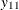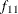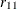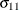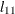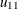2 date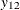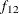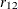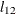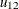Table 35.8: OUT= Data Set Continued

 Obs y2 FOR2 RES2 STD2 LCI2 UCI2 1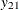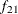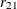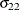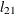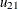2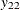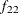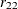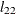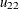Consider the following example:

proc varmax data=simul1 noprint;
id date interval=year;
model y1 y2 / p=1 noint;
run;

proc print data=out(firstobs=98);
run;


The output in Figure 35.66 shows part of the results of the OUT= data set for the preceding example.

Figure 35.66: OUT= Data Set

Obs date y1 FOR1 RES1 STD1 LCI1 UCI1 y2 FOR2 RES2 STD2 LCI2 UCI2
98 1997 -0.58433 -0.13500 -0.44934 1.13523 -2.36001 2.09002 0.64397 -0.34932 0.99329 1.19096 -2.68357 1.98492
99 1998 -2.07170 -1.00649 -1.06522 1.13523 -3.23150 1.21853 0.35925 -0.07132 0.43057 1.19096 -2.40557 2.26292
100 1999 -3.38342 -2.58612 -0.79730 1.13523 -4.81113 -0.36111 -0.64999 -0.99354 0.34355 1.19096 -3.32779 1.34070
101 2000 . -3.59212 . 1.13523 -5.81713 -1.36711 . -2.09873 . 1.19096 -4.43298 0.23551
102 2001 . -3.09448 . 1.70915 -6.44435 0.25539 . -2.77050 . 1.47666 -5.66469 0.12369
103 2002 . -2.17433 . 2.14472 -6.37792 2.02925 . -2.75724 . 1.74212 -6.17173 0.65725
104 2003 . -1.11395 . 2.43166 -5.87992 3.65203 . -2.24943 . 2.01925 -6.20709 1.70823
105 2004 . -0.14342 . 2.58740 -5.21463 4.92779 . -1.47460 . 2.25169 -5.88782 2.93863